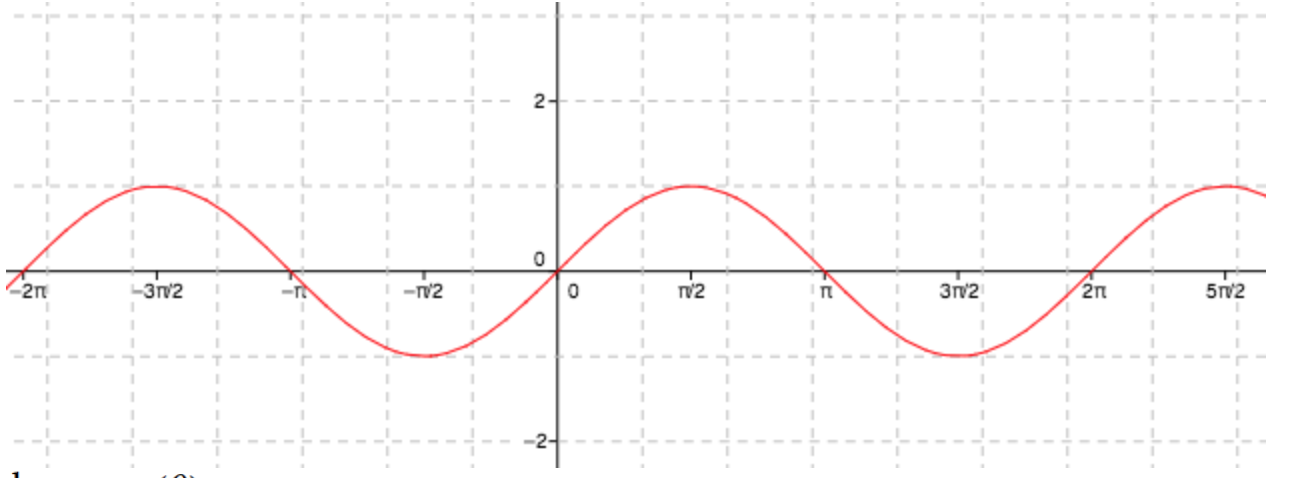### Home > A2C > Chapter 13 > Lesson 13.1.1 > Problem13-16

13-16.

If $π < θ <\frac { 3 \pi } { 2 }$, what must be true about the sign of:

Graph each function using the input bar below or on your graphing calculator. What do you observe about the $y$-values when $θ$ is between $π$ and /2?

When graphing using the input bar, sin($θ$) is equivalent to sin($x$).

1. sin(θ

The $y$-values are negative.2. cos(θ

Use the same method as part (a). It might also help to think about how the sin($x$) and cos($x$) graphs relate to each other.

3. tan(θ

Use the same method as part (a).

positive

4. $\frac { 1 } { \operatorname { cos } ( \theta ) }$

Does taking the reciprocal of something change its sign?